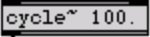# Digital Music Programming: Programming Lab 3 Making an Oscillator

This lab demonstrates how to create a sinewave generating program in C/C++.

in Max/MSP, the cycle~ object is an oscillator which generates a sinewave as an output. It takes two inputs: (1) the frequency in Hertz of the sinewave, and (2) the phase of the sinewave. Here is a cycle~ object with the default frequency being 100 Hz:Actually cycle~ is an interpolated wavetable which happens to have one cycle of a cosine in the contents of a 512 sample wavetable. But we will not worry about that.

An oscillator is generated from a sinewave which has the following mathematical equation:

```   cycle(frequency, phasor)
= cos(2 * pi (frequency * time + phasor))
= cos(2 * pi * frequency * time + phase)
```

How can the cosine function be implemented as an oscillator which takes frequency and phase input? The phase increment must be calculated from the frequency like this:

```    phase_increment = 2 * pi * frequency / sample_rate

```
This value must then be added to the previous phase_position in the cosine cycle for each unit of time. Here is a C for-loop which can be used to calculate the oscillator:

 ``` sample_count = 44100; // one second of sound amplitude = 0.5; sample_rate = 44100.0; frequency = 440.0; initial_phase = 0.0; sum = initial_phase; for (i=0; i

# Exercises

1. Write a command-line program which generates a sinewave soundfile using the above code example as a guideline. Use the code from programming lab 2 as a program template.

```